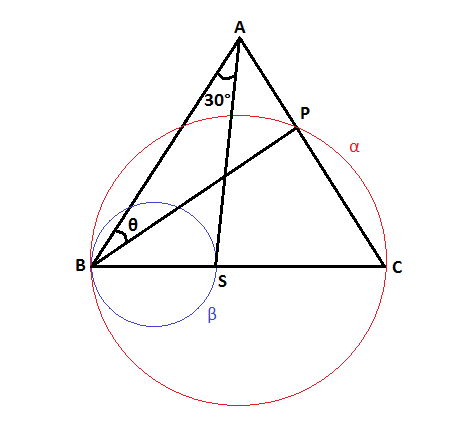# A geometry problem by Leonardo Joau

Geometry Level 5$ABC$ is an isosceles triangle with $\overline{AB}=\overline{AC}$.

$\alpha$ and $\beta$ are two circumferences that have centres in $\overline{BC}$ and intersects this straight line in points $B$,$S$ and $C$.

The diameters of $\alpha$ and $\beta$ are $\sqrt{2}+1$ and $1$, respectively.

If $\measuredangle{BAS}=30^\circ$ find $\angle{ABP}$.

Enter your answer in degrees.

Note: Image drawn not up to scale.

×

Problem Loading...

Note Loading...

Set Loading...